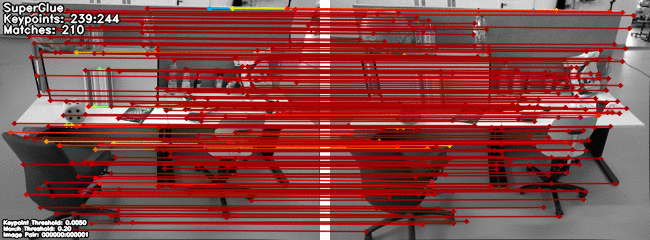1911.11763.pdf# 1. Introduction

Learning feature matching can be treated as a linear assignment problem, and can be further relaxed into an optimal transport problem

Linear Assignment: Given two sets, A and T, of equal size, together with a weight function $C: A \times T \rightarrow \mathbb{R}$. Find a bijection $f : A \rightarrow T$ such that the cost function is minimized:Quadratic Assignment: Given two sets, P ("facilities") and L ("locations"), of equal size, together with a weight function $w: P \times P \rightarrow \mathbb{R}$ and a distance function $d : L \times L \rightarrow \mathbb{R}$. Find the bijection $f: P \rightarrow L$ ("assignment") such that the cost function:Optimal Transport Problem: Generalization of the above linear assignment problem but sets are probability density functions, and the assignment is continuous. This makes the problem differentiable.

The cost function for optimal transport to be minimized is predicted by the Graph Neural net (GNN). The GNN uses self-attention (within image) and cross-attention (inter-image) to use both spatial and visual features of the keypoints. The Method is trained end-to-end from image pairs.

# 2. Superglue Architecture

2D Keypoints are generally projections of salient 3D points, like corners or blobs. This results in two constraints:

1. A keypoint can have at most a single correspondence in the other image
2. Some keypoints will not be matched owing to occlusions or detector failure

Formulation: Given two images A and B, each with sets of keypoints $p$, and associated visual descriptor $d$, call $(p, d)$ **as local features. Keypoints consist of $(x, y, c)$ **for position and detection confidence respectively. Visual descriptors $d_i \in \mathbb{R}^D$ can be obtained from CNNs or traditional descriptors

Partial Assignment: Owing to constraints 1 and 2. We have a partial assignment problem. Define a partial soft assignment matrix $\mathbf{P} \in [0, 1]^{M \times N}$ such that $\mathbf{P1}_N \leq \mathbf{1}_M$ and $\mathbf{P}^\top\mathbf{1}_M \leq \mathbf{1}_M$.

Goal is to design a NN to predict the assignment matrix from two sets of local features.

## 2.1 A short primer on Graph Neural Networks

The target of GNN is to learn a state embedding $\mathbf{h}_v \in \mathbb{R}^s$ which contains the information of the neighborhood for each node. The state embedding $\mathbf{h}_v$ is an s-dimension vector of node $v$ and can be used to produce an output $\mathbf{o}_v$ such as the node label.